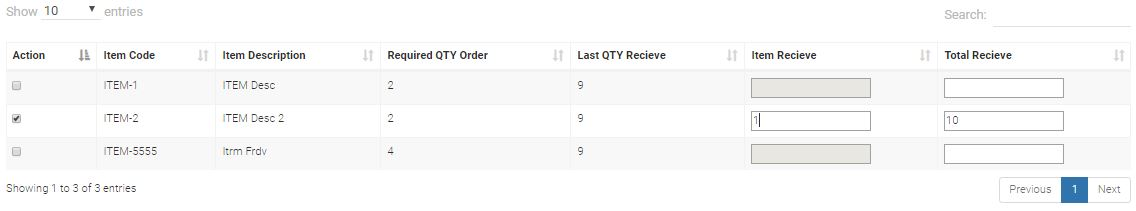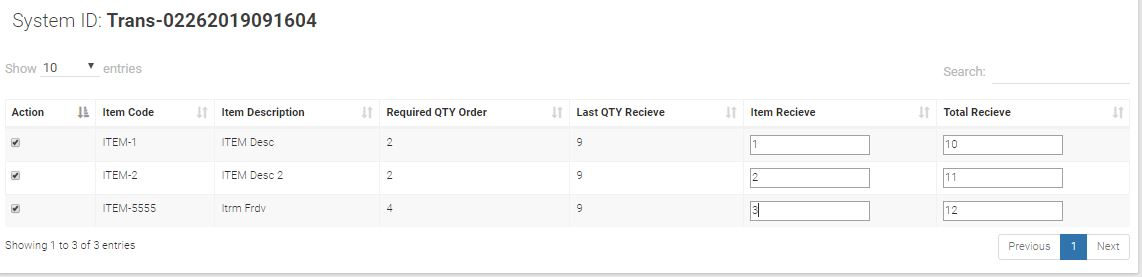# #StackBounty: #php #codeigniter Values from textbox array not updating

### Bounty: 50

Im new in this forum and i’m having a hard time updating selected value from checkbox coming from the textbox value.

Scenraio:

I want to update selected items, For example I want to update Item-2 and the textbox from item recieve will be enable, I will enter a number for example 1 and the textbox from total receive will automatically sum using ajax.

The problem is after submiting the value from total recieve the records that was updating is blank, but when I try to check and print_r the value is there.

And one more thing if all checkbox are all checked and enter a number for each item recieve, the value that it only get is the last value and will be updated will all selected checkbox.

Note:
Checkbox is an array
Textbox from total recieve is an array

Here’s my UI:Controller:

``````public function recitem_insert(){

\$check = \$this->input->post('check');
\$total_rec = \$_POST['total_rec'];

if(isset(\$check)){ //Check if check is checked

for(\$i=0;\$i<sizeof(\$check);\$i++){
for(\$j=0;\$j<sizeof(\$total_rec);\$j++){

\$updateData = array('rec_qty' => \$total_rec[\$j] );
\$this->dbquery->modUpdatedynamicval('tblstock', 'id', \$updateData, \$check[\$i]);

}
}//end for loop

echo "
window.close();
";

}else{ //End If
}

}
``````

View:

``````<form method="post" action="<?php echo base_url() ?>user/recitem_insert">

``````

System ID:

db->query("SELECT * FROM tblstock where process_id = '\$process_id'");
foreach (\$query->result() as \$row){
?>

rec_qty;  ?>">

Action
Item Code
Item Description
Required QTY Order
Last QTY Recieve
Item Recieve
Total Recieve

id; ?>" onclick="valueChanged()">
item_code; ?>
description; ?>
qty_order; ?>
rec_qty; ?>

<!-- /.box-body -->
</div>

</form>
``````

Model:

``````public function modUpdatedynamicval(\$table, \$column, \$data, \$equal_to){

\$this->db->where(\$column, \$equal_to);
\$this->db->update(\$table, \$data);

}
``````

Any help will be gladly appreciate.

Thank you..

EDIT:

Lets assume,
I’ve input 1 in item_receive textbox one and the total recieve will be 10,
2 in item_receive textbox two and the total recieve will be 11,
3 in item_receive textbox three and the total recieve will be 12,CODE:

``````\$check = \$this->input->post('check');
\$total_rec = \$_POST['total_rec'];

echo 'Check Value';
print_r(\$check);

echo '<br><br>';

echo 'Total Recieve';
print_r(\$total_rec);
``````

OUTPUT:

``````Check ValueArray (  => 1  => 2  => 3 )

Total RecieveArray (  => 10  => 11  => 12 )
``````

But If I only inout the second textbox here’s the output:

``````Check ValueArray (  => 2 )

Total RecieveArray (  =>  => 11  => )
``````

Get this bounty!!!

This site uses Akismet to reduce spam. Learn how your comment data is processed.